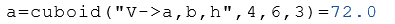# Class Cuboid

Calculation of the properties of cuboids

## Description

The class Cuboid calculates properties of a cuboid.

## Properties

The following properties can be queried as a result or passed as an argument.

#### Description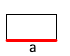a Length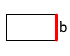b Width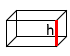h Height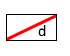d Diagonal of the base (a x b)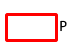P Perimeter of the base (a x b)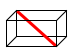e Space diagonal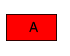A Area of the base (a x b)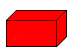S Surface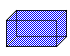V Volume

## Syntax

Object = Cuboid ("arg names", arguments)

Value = Cuboid ("get -> arg names", arguments)

## Example

Calculation of the properties of the side lengths $$a$$ and $$b$$ and the height $$h$$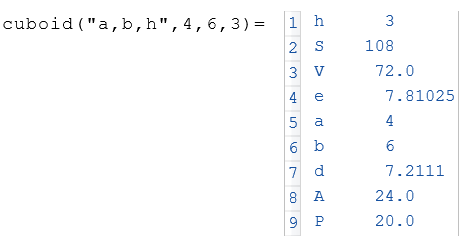The initialized object $$a$$ displays the volume $$V$$.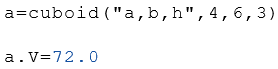From the side lengths $$a$$ and $$b$$ and the height $$h$$ the volume $$V$$ is calculated.